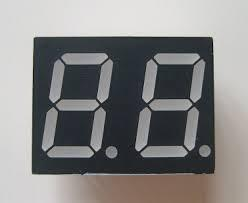# Two-digit number

I am a two-digit number less than 20. When I divided by three, then you get the rest 1 and when you divide me by four you get also rest 1. What number am I?

x =  13

### Step-by-step explanation:Did you find an error or inaccuracy? Feel free to write us. Thank you!## Related math problems and questions:

• Divisible by nineHow many three-digit natural numbers in total are divisible without a remainder by the number 9?
• Twenty-fiveHow many are three-digit natural numbers divisible by 25?
• Digit sumFind the smallest natural number n, the digit sum of which is equal to 37.
• Four-digit numberFind also a four-digit number, which quadrupled written backwards is the same number.
• How manyHow many numbers are less than 222 with a digit sum is 8?
• PlumsIn the bowl are plums. How many would be there if we can divide it equally among 8, 10 and 11 children?
• NumberWhat number should be placed instead of the asterisk in number 702*8 to get a number divisible by 6?
• Two-digit numberDigit sum of thinking two-digit natural number is 11. When it exchanging a sequence of digits, given a number which is 27 less than the thinking number. Find out which number I think.
• DivisibilityDetermine the smallest integer which divided 11 gives remainder 4 when divided 15 gives remainder 10 and when divided by 19 gives remainder 16.
• Sports studentsThere are 120 athletes, 48 volleyball players, and 72 handball players at the school with extended sports training. Is it possible to divide sports students into groups so that the number in each group is the same and expressed by the largest possible num
• Prime factorsWrite 98 as a product of prime factors
• GroupsIn the 6th class there are 60 girls and 72 boys. We want to divide them into groups so that the number of girls and boys is the same. How many groups can you create? How many girls will be in the group?
• Prime divisorsFind 2/3 of the sum's ratio and the product of all prime divisors of the number 120.
• Unknown integerFind the smallest integer: divided by 2, the remainder is 1. divided by 3, the remainder is 2. divided by 4, the remainder is 3. ... divided by eight, the remainder is 7, divided by 9 the remainder is 8.
• PIN codePIN on Michael credit card is a four-digit number. Michael told this to his friend: • It is a prime number - that is, a number greater than 1, which is only divisible by number one and by itself. • The first digit is larger than the second. • The second d
• Number with onesThe first digit of the number is 1, if we move this digit to the end we get a 3 times higher number, which is the number?
• A number 5A number is divisible by 24, 25, and 120 if it is increased by 20. Find the number.